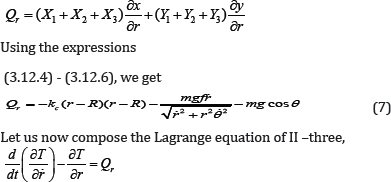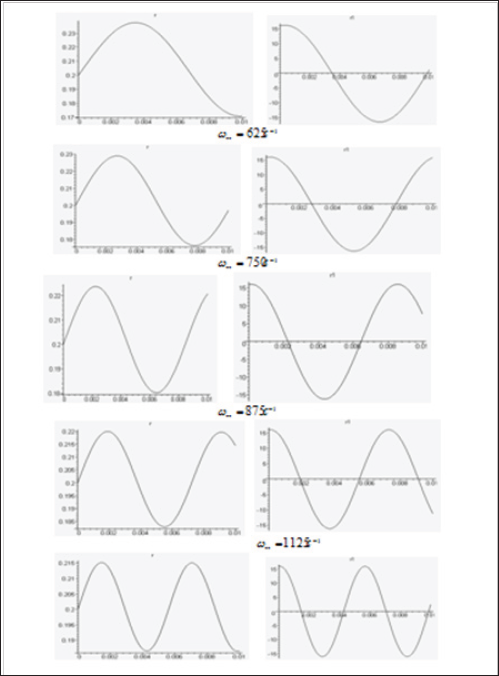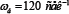## Friday, 12 March 2021

### Lupine Publishers | Theoretical Study of Movements Trash in Cotton Lobules In Cleaner Small and Large Trash

Lupine Publishers | Journal of Textile and Fashion Designing

## Abstract

The article considered mathematical model that describes the separation of large and small trash by weight of cotton, with elastic connection on the cotton. The relationship between the forces acting on the speck on the angular velocity and the radius of the working drum cleaner, laws of motion specks obtained on the surface and inside the cotton mass on the process parameters.

Keywords: Pulp; Speck; Elastic element; The force of the bunch; The dry friction force; Kolkova drum; Surface netted; The angular velocity

## Introduction

Known designs of cleaners are based on shock-shaking effects on fibrous material . Despite the fact that improving the design of cleaners has an ever-increasing trend, the industry's demand for highly efficient cleaners does not decrease. It should also be noted that, with the improvement of the designs of cleaners, the basic working elements of them remain the same. This, in our opinion, is a consequence of the fact that the essence of the process of cleaning fibrous material from weedy impurities is not sufficiently disclosed, the available dynamic and mathematical models do not fully correspond to the actual cleaning situation. Therefore, any progressive attempts to describe the purification process using the latest achievements of science should be welcomed. Linear , nonlinear  dynamic models of the process for cleaning fibrous material are known. Also developed are models aimed at isolating weedy impurities from the surface of a layer of fibrous material , isolating weed particles from the fibrous material by shearing the layers , isolating the litter during their interaction with the working organs of the disintegrated .

There are also attempts to describe the process by empirical formulas based on experimental material . Despite the fact that the models adequately solve the tasks assigned to them, due to accepted assumptions in the development or in the analysis of models, some questions were not answered, which served as the development of a dynamic model that takes into account the masses of the mote associated with fibrous material by an elastic bond, interacting with the surface of the material by the force of dry friction. Let the fibrous mass contain a mote with a mass that is connected to it by an elastic element and by the force of dry friction. Depending on the location in the composition of the pulp, we divide the trash into two types. The first type of trash is located on the surface of the scrap and is in contact with the mesh surface. Such mote has an angular velocity equal to the speed of the spike, and in addition, under the action of a centrifugal force, they preferentially move through the opening of the mesh in the radial direction from the center.

The distance from the center to the mote will be denoted by, we assume that the mote is connected to the fibrous mass through an elastic element with variable rigidity and write down the equation of motion of the mote.Where - coefficient of stiffness of a bundle of a mote with fibers and depends on the displacement r -R , T.e. kc = kc(r -R) , where function k (z) is determined experimentally and has a monotonically decreasing character, and after detachment of the mote from the fibrous mass, it vanishes, i.e. should be taken kc (z) = 0 at z > z0 . In particular, this function can be chosen in the form of a piecewise constant k = k0 at z < z0 and k = 0 at z > z0 or piecewise linear k = kx (z" - z) at z z(| and k = 0 at z ≥ z0 Moving r(t) satisfies the following initial conditions r = R, r = Rωα2 at t = 0 In this case, the leaving time t = tk the mote of the mesh surface is determined from equations:

k[r (tk) - R] = 0

If to ≤ tk then the mote has time to leave the surface of the grid during the contact of the scrap with the surface of the grid, but if to > tk , then the mote after the interaction of the scrap with the reticulate surface does not leave the scrap and continues to move with it. The results of integrating equations (1) are presented in Figure 1. The second type includes the mote, which is in the composition of the pulp at a distance from the center of the drum, and r1 < R . In this case, these kinds of trash move for some time in the region within the fibrous mass, the movement of the trash relative to the scrap preferably in the radial direction [8-10]. To compose the equation of motion of the mote, we assume that the force of the bond between the mote and fibers acts on the mote, and also the frictional force at the surface of their contact as a result of the movement of the mote relative to the fibrous mass.

Figure 1: Obligations of radial displacement mote for time for different values of movement angular velocity of the drum.By supplying the expressions for the kinetic energy (3) and the generalized force (7), we form the equation for the definition of the variable r .On Figure 2 the curves of the distance dependence r(t) (m) and the rate of moth removal r(t) (m/c) in the case when θ = ωbt , kc = kc0 = const Calculations were made for different values of the parameter ω** =√kc /mc (1/cek) ? With small masses of mote,this value can assume large values. Therefore, calculations were carried out for ω** > 500 1/ cek. The calculations also R = 0.2 , ωb = 80 1/cek, f = 0.3 O= 200,√k/m = 801/cek

Figure 2: Dependence of displacement r(t) and speed r1 = r (e) of time for different values of the parameter ω**(c-1 )It can be seen from the results that this parameter plays a significant role in the process of removing the mote . At small values, the mote is rapidly removed from the scrap, for example, if it is assumed that the detachment of the mote from the scrap occurs when the stretch δ = 0.02, then the weight of the mote for which the parameter a, takes on values ω** < 1000 then they are removed from the pulp. Other specks where ω** > 1000 , then the initial velocity of the mote will be insufficient to remove from the mass. If the speed of the drum is takenthen specks with a parameter ω** = 1000 will also be deleted.

## Conclusion

As a result of the analysis of the dynamic model for the interaction of weed impurities with the pulp with an elastic coupling between them, the dependency of the parameters of the displacement of the rubbish on the angular velocity and the radius of the cleaning drum of the cleaner is established, which can be used in the development of purifiers for raw cotton.